Novice, Paragon, Master

# npm

## @jwalsh/stochastic

0.2.4 • Public • Published

# stochastic

## Install

`npm install @jwalsh/stochastic --save`

## Examples

Real-world examples will be provided from some of the following texts or courses:

• 18.445 Introduction to Stochastic Processes
• 6.041 / 6.431 Probabilistic Systems Analysis and Applied Probability
• Introduction to Stochastic Processes, Erhan Cinlar
• Stochastic Modeling: Analysis & Simulation, Barry L. Nelson
• Markov Models: An Introduction to Markov Models, Steven Taylor

## Usage

### ES2015

```import * as stoch from '@jwalsh/stochastic';
const exp = stoch.exp(20);```

### CommonJS

```var stoch = require('@jwalsh/stochastic');
var norm = stoch.norm(1, 1, 100);```

### CDN

```<script src="https://cdn.rawgit.com/jwalsh/stochastic/master/dist/bundle.min.js"></script>
<script>
console.log(stoch.exp(20));
</script>```

## API

### poissP

Returns an array with the times of each arrival in a Poisson Process with rate `lambda` until time `T`.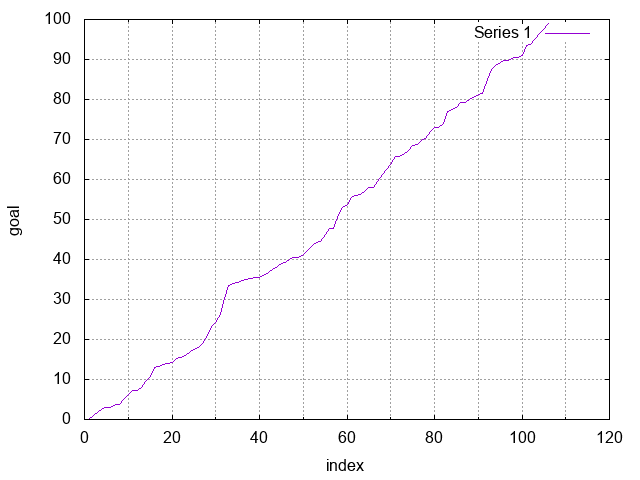Exercise: Assuming you get 10 emails per hour over the course of an 8 hour day, what's the distribution of the number of emails you receive over the course of a standard 261 work-day year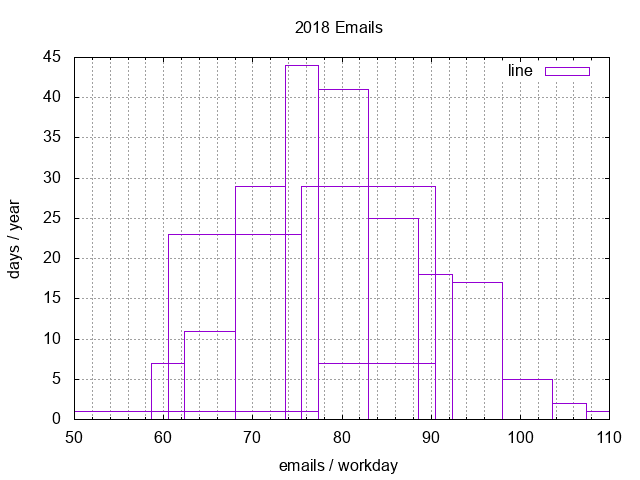Parameters

• `lambda` number (rate) (optional, default `0`)
• `T` number time as positive number (optional, default `0`)
• `path` boolean (optional, default `true`)

Examples

`const poissP = stoch.poissP(1, 100, true);`
`const emails = stoch.hist(Array(261).fill().map(e => stoch.poissP(10, 8, true).length));`

Returns Array<number> times of each arrival in a Poisson Process

### average

Returns the average.

Parameters

• `data`
• `values` Array<number>

Examples

`const avg = stoch.average([1, 2, 3]);`

Returns number standard deviation as positive number

### std

Returns the standard deviation.

Parameters

Examples

`const std = stoch.std([2, 3, 4, 4, 4, 5, 6]);`

Returns number standard deviation as positive number

### mock

Returns a mock data set that uses the same standard deviation and average.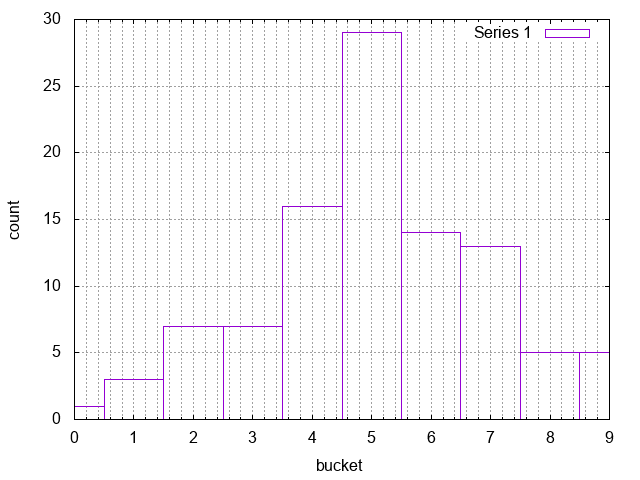Parameters

• `values` Array<number>
• `num` number a positive integer (optional, default `1`)

Examples

`const mock = stoch.mock(stoch.norm(1, 1, 100));`

Returns number standard deviation as positive number

### norm

Returns an array with `num` normal random variables in a normal distribution of mean `mu` and standard deviation `sigma`.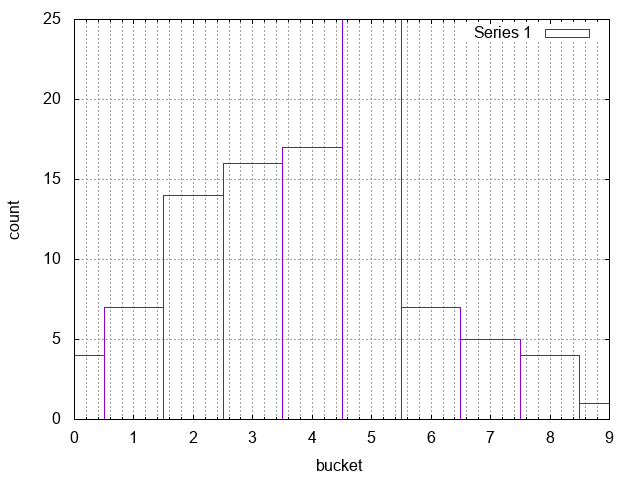Parameters

• `mu` number the mean or expectation of the distribution (and also its median and mode) (optional, default `1`)
• `sigma` number standard deviation as positive number (optional, default `0`)
• `num` number a positive integer (optional, default `1`)

Examples

`const norm = stoch.norm(1, 1, 100);`

Returns Array<number> normal random values

### brown

Returns an array corresponding to the path of Brownian motion from time 0 to `T` with drift parameter `mu` and volatility parameter `sigma` (the process is initialized to be 0). The i-th entry in the array corresponds to the Brownian process at time `i * (T / steps)`.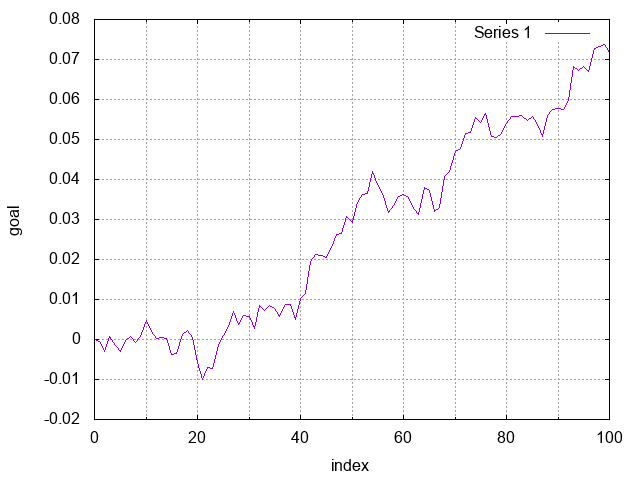Parameters

• `mu` number drift parameter (a real number)
• `sigma` number volatility parameter (strictly positive real)
• `T` number time (strictly positive real)
• `steps` number (positive integer)
• `path` boolean (optional, default `true`)

Examples

`const brown = stoch.brown(1.0, -0.1, +0.1, 100, true);`

Returns Array<number> Brownian motion path

### GBM

Returns an array corresponding to the path of geometric Brownian motion from time 0 to `T` with drift parameter `mu` and volatility parameter `sigma` (the process is initialized to be S0). The i-th entry in the array corresponds to the geometric Brownian process at time `i * (T/steps)`.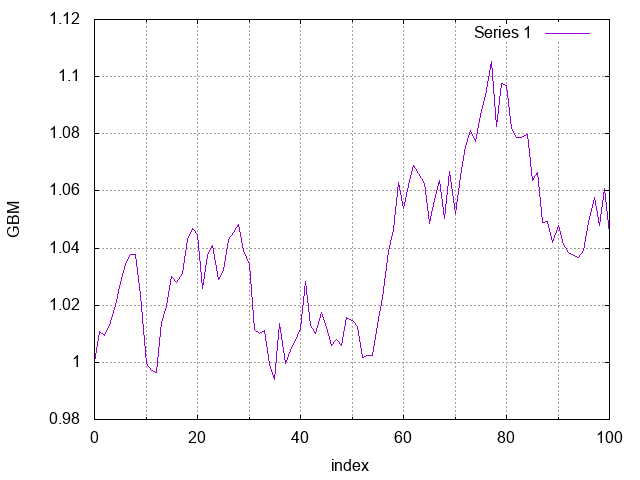Parameters

• `S0` number initialized process value
• `mu` number drift parameter
• `sigma` number volatility parameter (strictly positive real)
• `T` number time (strictly positive real)
• `steps` number (positive integer)
• `path` boolean (optional, default `true`)

Examples

`const GBM = stoch.GBM(1.0, -0.1, 0.1, 1.0, 100, true);`

Returns Array<number> geometric Brownian motion

### DTMC

Returns an array with the states at each step of the discrete-time Markov Chain given by `transMatrix` (a square matrix). The number of transitions is given by `steps`. The initial state is given by start (the states are indexed from 0 to n-1 where n is the number of arrays in transMatrix).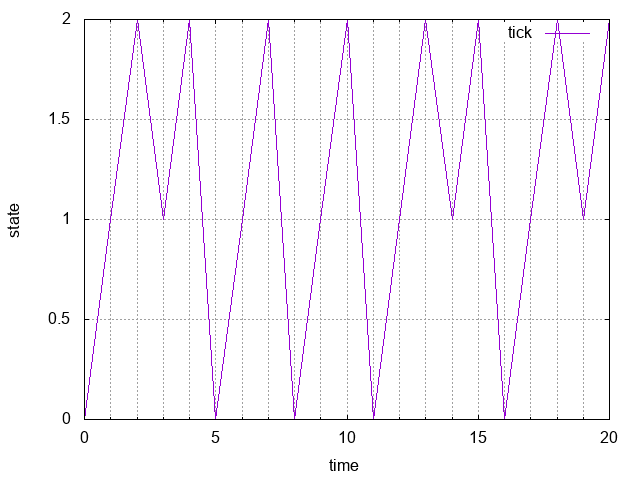Parameters

Examples

`const DTMC = stoch.DTMC([[0,1,0],[0,0,1],[1,0,0]], 20, 0, true);`

Returns Array<number>

### collate

Returns the `transMatrix` for an array of mapped `states` to numerical values.

Parameters

Examples

`const collate = stoch.collate([0,1,0,0,0,1,1,0,0]);`

Returns Array<Array<number>> transMatrix

### CTMC

Returns an object with the {key:value} pair {time:state} at each step of the continuous-time Markov Chain given by transMatrix (a square matrix). The Markov Chain is simulated until time `T`. The initial state is given by `start` (the states are indexed from 0 to n-1 where n is the number of arrays in `transMatrix`).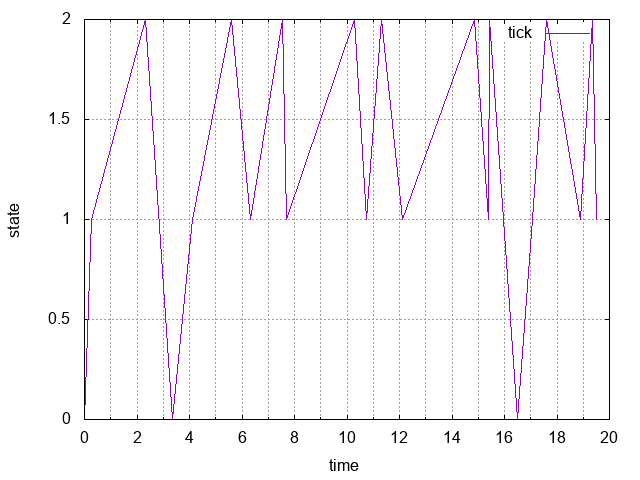Parameters

Examples

`const CTMC = stoch.CTMC([[0,1,0],[0,0,1],[1,0,0]], 20, 0, true);`

Returns Object Continuous-time Markov chain

### sample

Generates a random sample (with replacement) from array `arr` of observations. Number of observations `n` is specified by the user.

Parameters

Examples

`const sample = stoch.sample([1,2,3,4,5], +10);`

Returns Array<number> random sample

### exp

Generates an exponential random variable with rate parameter `lambda`.

Parameters

• `lambda` number (positive) (optional, default `1`)

Examples

`const exp = stoch.exp(20);`

Returns number variable

### pareto

Generates a Pareto random variables with parameters `x_m` and `alpha`.

Parameters

• `x_m`
• `alpha` number
• `x_a` number (positive)

Examples

`const pareto = stoch.pareto(+20.0, -1.0);`

Returns number distribution

### hist

Generates a histogram object from an array of data. Keys denote the lower bound of each bin and the values indicate the frequency of data in each bin.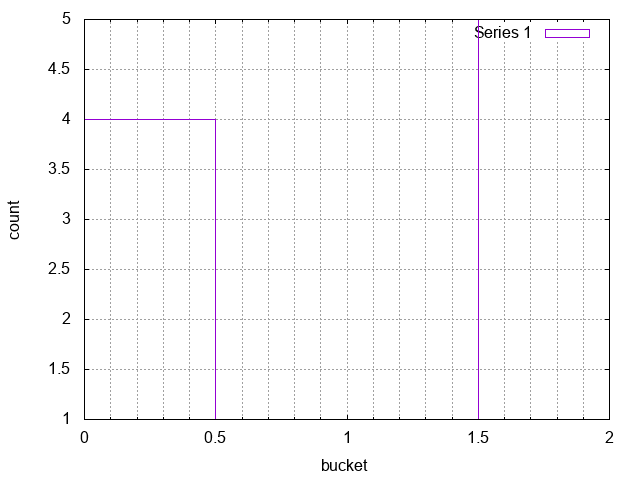Parameters

Examples

`const hist = stoch.hist([1,1,1,1,2,3,3,4,4,4]);`

Returns Object histogram

## Development

Testing is provided with the JavaScript implementation of http://clojure.org/guides/spec in `tests/`.

The MIT License (MIT)

Copyright (c) 2014 Nathan Epstein

Permission is hereby granted, free of charge, to any person obtaining a copy of this software and associated documentation files (the "Software"), to deal in the Software without restriction, including without limitation the rights to use, copy, modify, merge, publish, distribute, sublicense, and/or sell copies of the Software, and to permit persons to whom the Software is furnished to do so, subject to the following conditions:

The above copyright notice and this permission notice shall be included in all copies or substantial portions of the Software.

THE SOFTWARE IS PROVIDED "AS IS", WITHOUT WARRANTY OF ANY KIND, EXPRESS OR IMPLIED, INCLUDING BUT NOT LIMITED TO THE WARRANTIES OF MERCHANTABILITY, FITNESS FOR A PARTICULAR PURPOSE AND NONINFRINGEMENT. IN NO EVENT SHALL THE AUTHORS OR COPYRIGHT HOLDERS BE LIABLE FOR ANY CLAIM, DAMAGES OR OTHER LIABILITY, WHETHER IN AN ACTION OF CONTRACT, TORT OR OTHERWISE, ARISING FROM, OUT OF OR IN CONNECTION WITH THE SOFTWARE OR THE USE OR OTHER DEALINGS IN THE SOFTWARE.

## Keywords

### Install

`npm i @jwalsh/stochastic`

### Repository

github.com/jwalsh/stochastic